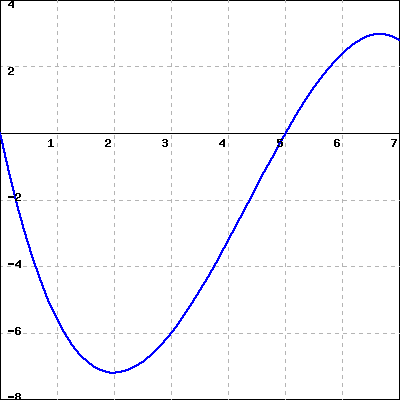Consider the graph of the function $f(x)$ shown below.(Click on the graph for a larger version)

A. Estimate the integral
$\int_0^7{f(x)dx} \approx$

B. If $F$ is an antiderivative of the same function $f$ and $F(0) = 50$, estimate $F(7)$:
$F(7) \approx$